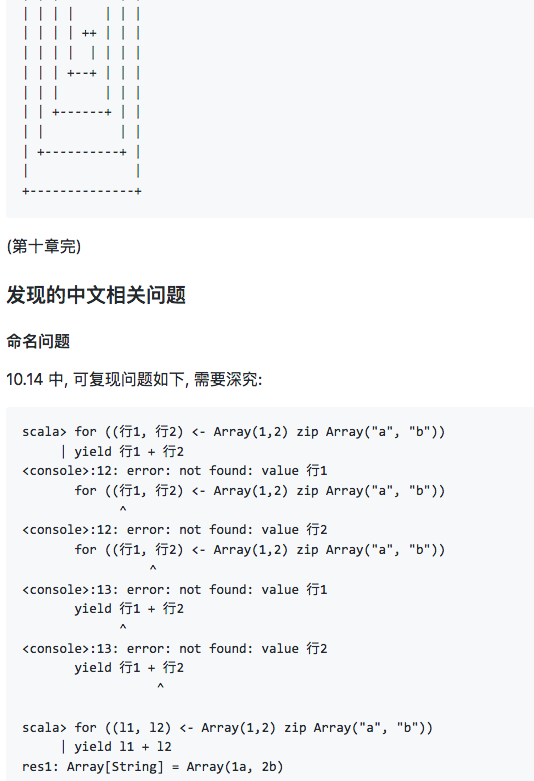## 疑似中文命名bug

``````scala> for ((行1, 行2) <- Array(1,2) zip Array("a", "b"))
| yield 行1 + 行2
for ((行1, 行2) <- Array(1,2) zip Array("a", "b"))
^
for ((行1, 行2) <- Array(1,2) zip Array("a", "b"))
^
yield 行1 + 行2
^
yield 行1 + 行2
^

scala> for ((l1, l2) <- Array(1,2) zip Array("a", "b"))
| yield l1 + l2
res1: Array[String] = Array(1a, 2b)``````

## 第九章 控制抽象

``````  def 文件匹配(查询: String, 匹配器: (String, String) => Boolean) =
for (文件 <- 此处文件; if 匹配器(文件.getName, 查询))
yield 文件

def 文件名结尾(查询: String) =
文件匹配(查询, _.endsWith(_))

def 文件名包括(查询: String) =
文件匹配(查询, _.contains(_))

def 文件名正则匹配(查询: String) =
文件匹配(查询, _.matches(_))``````

## 第十章 组合和继承

``````import 元素类.元素

object 螺旋 {
val 空格 = 元素(" ")
val 角 = 元素("+")

def 螺旋(边数: Int, 方向: Int): 元素类 = {
if (边数 == 1)
元素("+")
else {
val 旋 = 螺旋(边数 - 1, (方向 + 3) % 4)
def 竖条 = 元素('|', 1, 旋.高度)
def 横条 = 元素('-', 旋.宽度, 1)
if (方向 == 0)
(角 在旁 横条) 在上 (旋 在旁 空格)
else if (方向 == 1)
(旋 在上 空格) 在旁 (角 在上 竖条)
else if (方向 == 2)
(空格 在旁 旋) 在上 (横条 在旁 角)
else
(竖条 在上 角) 在旁 (空格 在上 旋)
}
}

def main(参数: Array[String]) = {
val 边数 = 参数(0).toInt
println(螺旋(边数, 0))
}
}``````

``````\$ scala 螺旋 17
+----------------
|
| +------------+
| |            |
| | +--------+ |
| | |        | |
| | | +----+ | |
| | | |    | | |
| | | | ++ | | |
| | | |  | | | |
| | | +--+ | | |
| | |      | | |
| | +------+ | |
| |          | |
| +----------+ |
|              |
+--------------+ ``````

## 中文编程

在所有编程语言和领域中尝试编写中文代码，开发相关工具，总结经验，一致代码风格。包括中文命名，汉化现有语言，创造中文语法的编程语言等等。作为最熟悉的母语，用来编写代码会让代码更容易被自己和母语相同的其他开发者理解。基于英文的编程语言和框架中，使用中文命名有时有技术问题。希望这里为后人趟雷，填坑。多数现有API是英文的，这里也会对其中一些常用的进行汉化。当然，这里也会对基于中文的编程语言进行探讨。包括汉化基于英文的编程语言，以及创造新的编程语言。